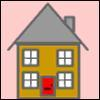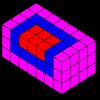# Resources tagged with: Square numbers

Filter by: Content type:
Age range:
Challenge level:

### There are 15 results

Broad Topics > Properties of Numbers > Square numbers### Square Routes

##### Age 11 to 14Challenge Level

How many four digit square numbers are composed of even numerals? What four digit square numbers can be reversed and become the square of another number?### Digat

##### Age 11 to 14Challenge Level

What is the value of the digit A in the sum below: [3(230 + A)]^2 = 49280A### Filling the Gaps

##### Age 14 to 16Challenge Level

Which numbers can we write as a sum of square numbers?### Factors and Multiples Puzzle

##### Age 11 to 14Challenge Level

Using your knowledge of the properties of numbers, can you fill all the squares on the board?### Four Coloured Lights

##### Age 11 to 14Challenge Level

Imagine a machine with four coloured lights which respond to different rules. Can you find the smallest possible number which will make all four colours light up?### Odd Differences

##### Age 14 to 16Challenge Level

The diagram illustrates the formula: 1 + 3 + 5 + ... + (2n - 1) = nÂ² Use the diagram to show that any odd number is the difference of two squares.##### Age 14 to 16Challenge Level

Robert noticed some interesting patterns when he highlighted square numbers in a spreadsheet. Can you prove that the patterns will continue?### Time of Birth

##### Age 11 to 14Challenge Level

A woman was born in a year that was a square number, lived a square number of years and died in a year that was also a square number. When was she born?### Sticky Numbers

##### Age 11 to 14Challenge Level

Can you arrange the numbers 1 to 17 in a row so that each adjacent pair adds up to a square number?### Generating Triples

##### Age 14 to 16Challenge Level

Sets of integers like 3, 4, 5 are called Pythagorean Triples, because they could be the lengths of the sides of a right-angled triangle. Can you find any more?### Iff

##### Age 14 to 18Challenge Level### Triangular Triples

##### Age 14 to 16Challenge Level

Show that 8778, 10296 and 13530 are three triangular numbers and that they form a Pythagorean triple.### Smith and Jones

##### Age 14 to 16Challenge Level

Mr Smith and Mr Jones are two maths teachers. By asking questions, the answers to which may be right or wrong, Mr Jones is able to find the number of the house Mr Smith lives in... Or not!### Triangles Within Squares

##### Age 14 to 16Challenge Level

Can you find a rule which relates triangular numbers to square numbers?### Summing Squares

##### Age 14 to 16Challenge Level

Discover a way to sum square numbers by building cuboids from small cubes. Can you picture how the sequence will grow?